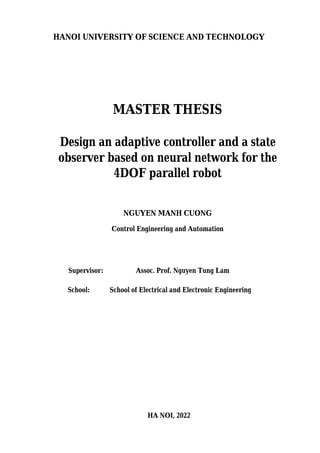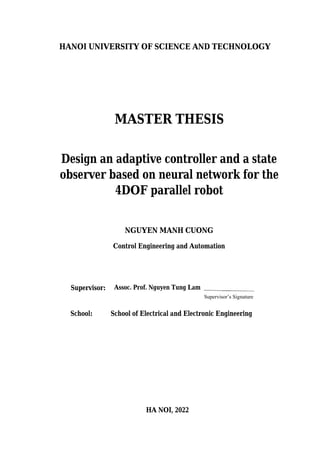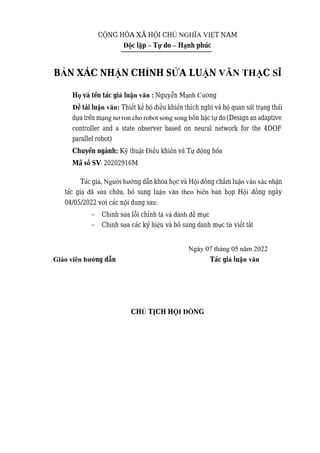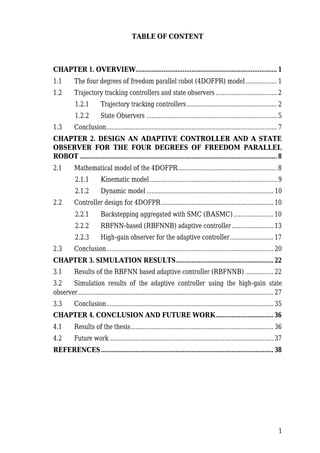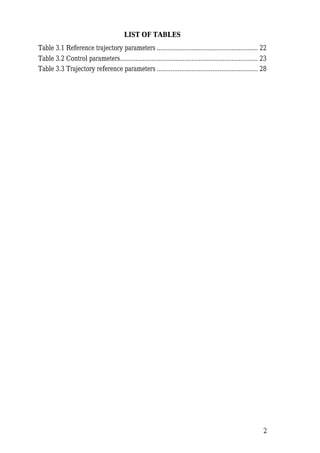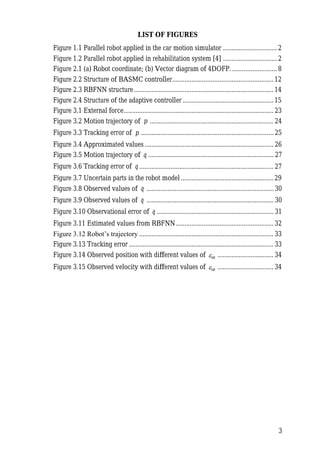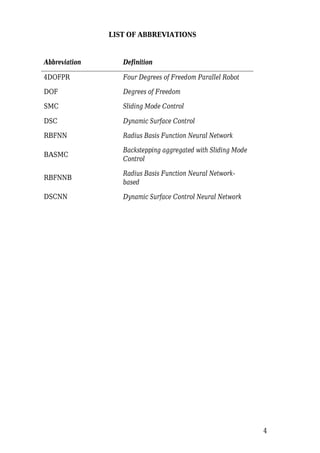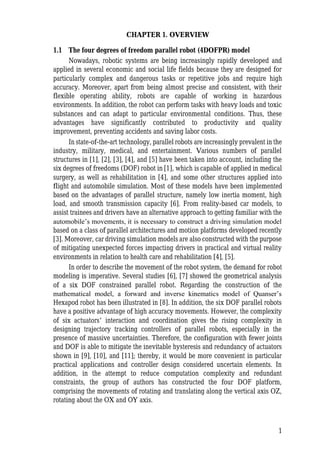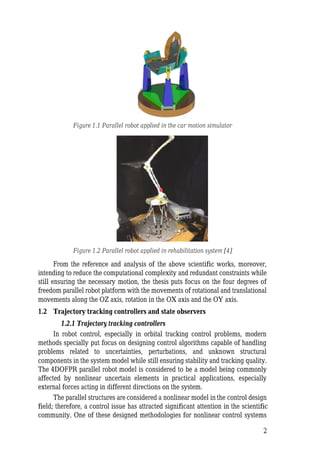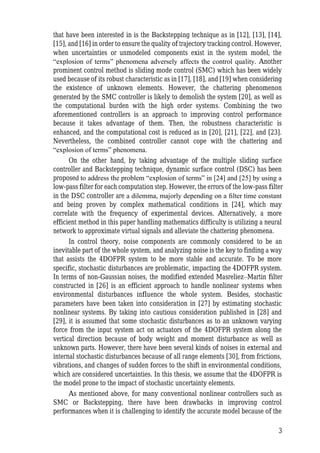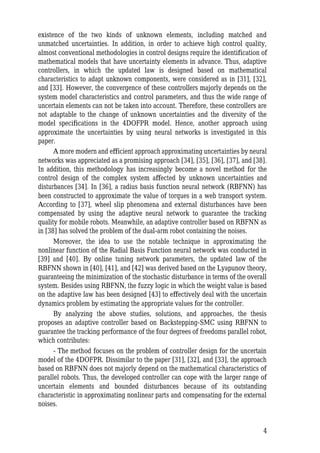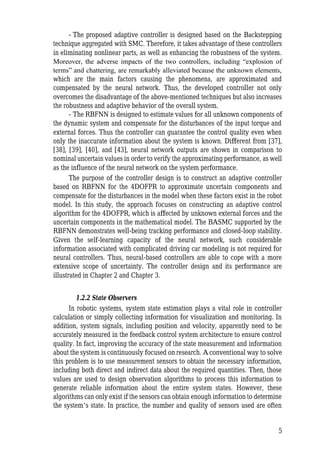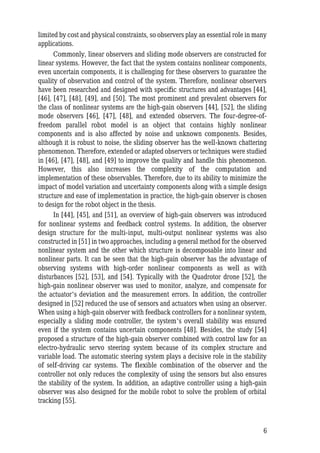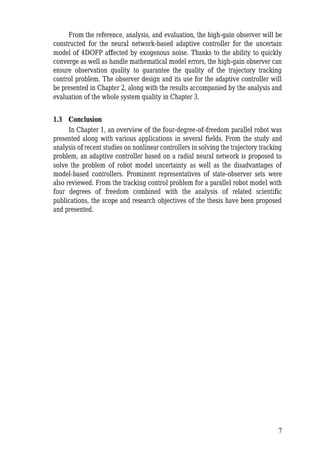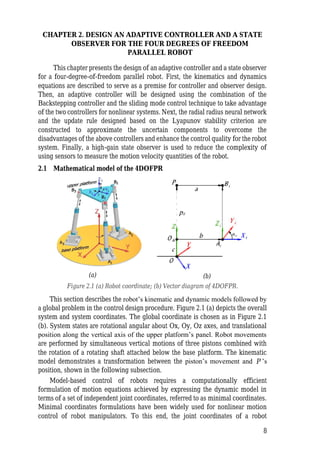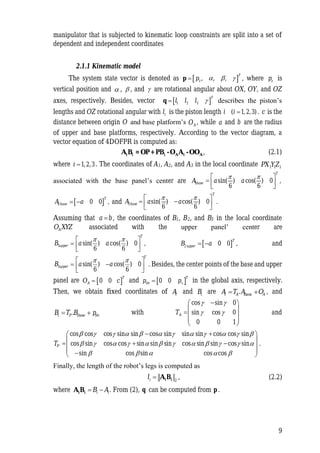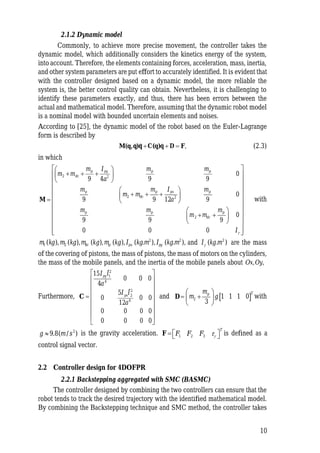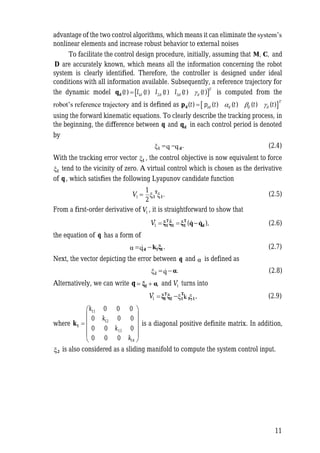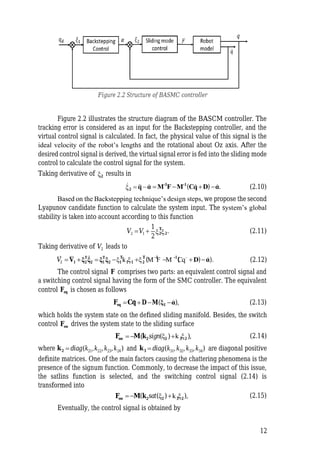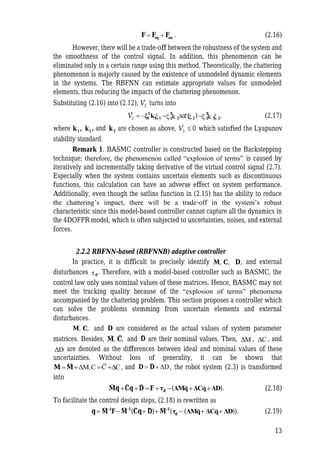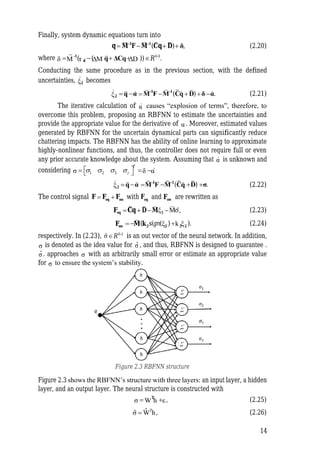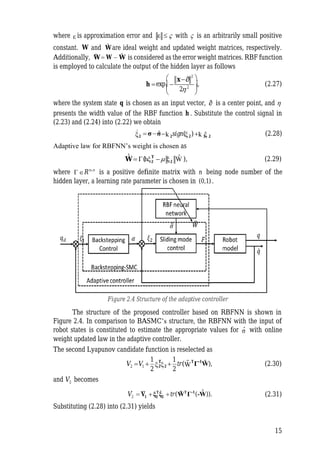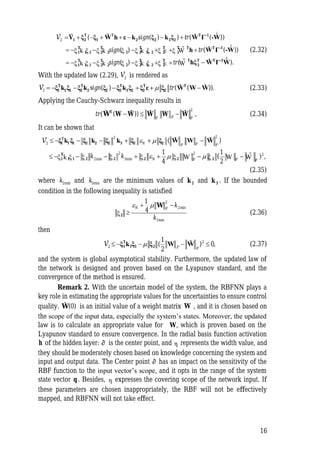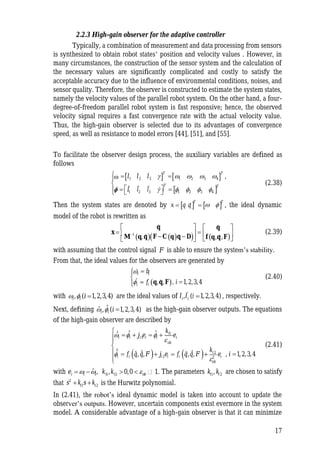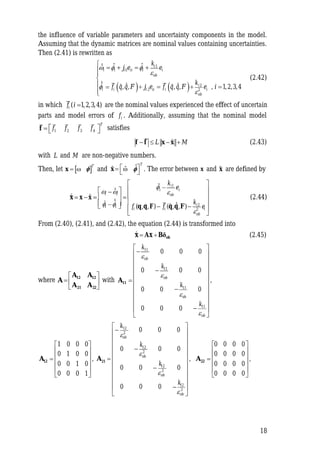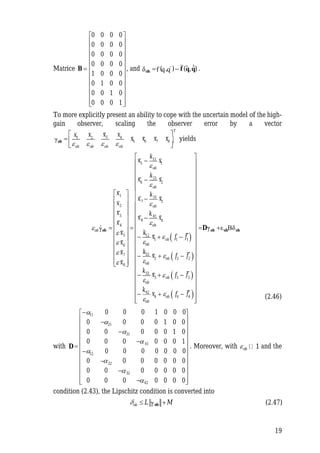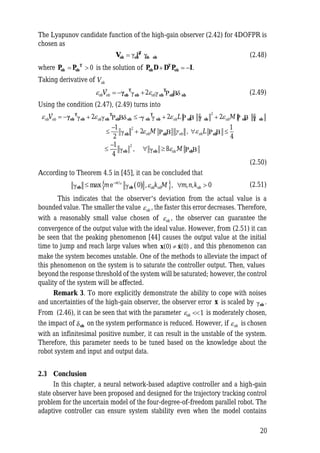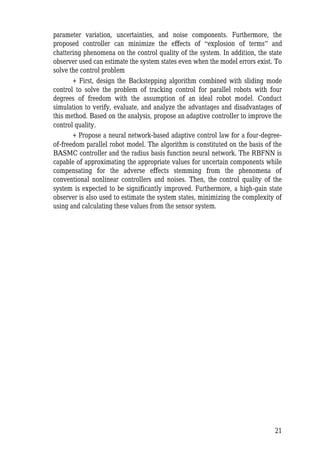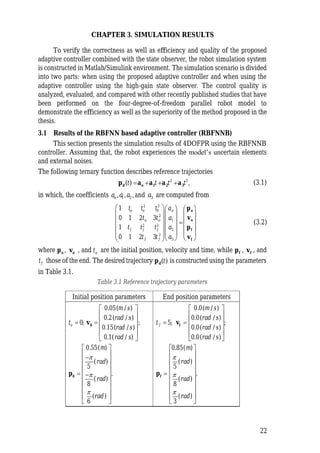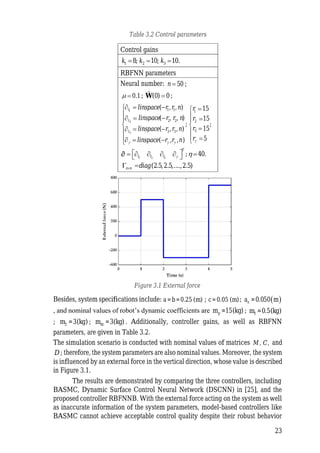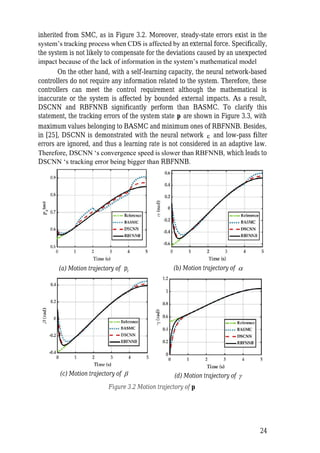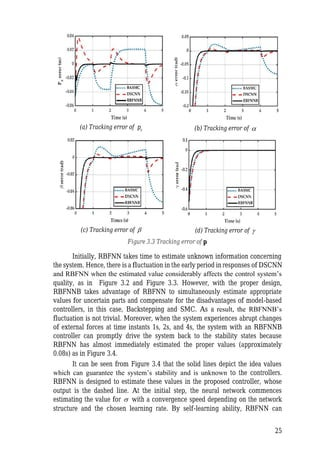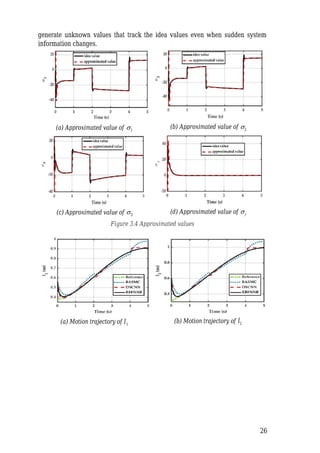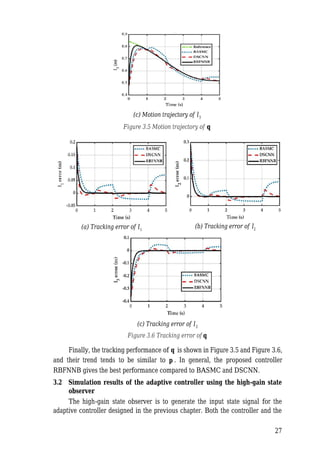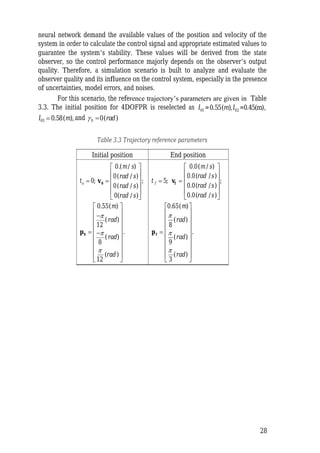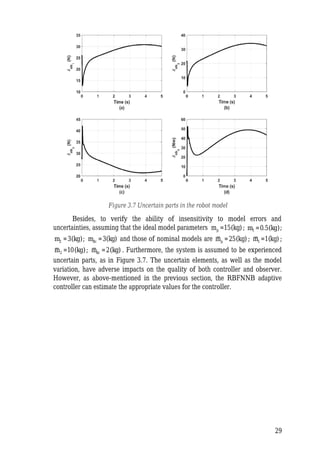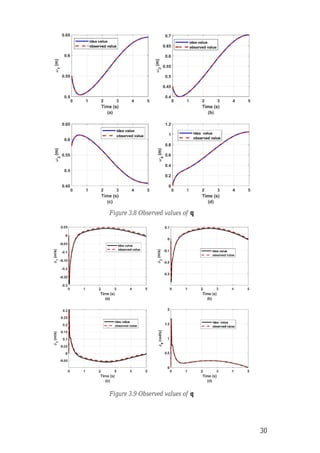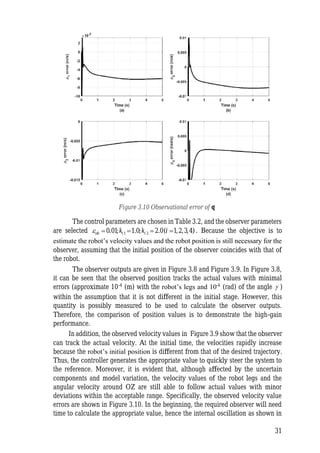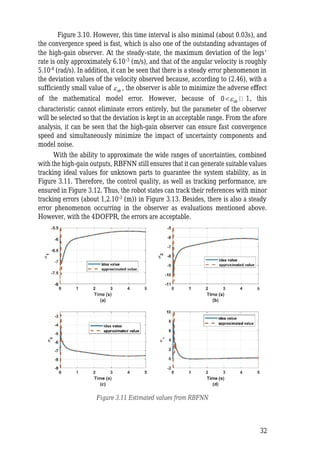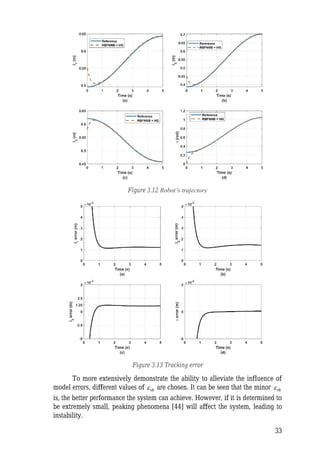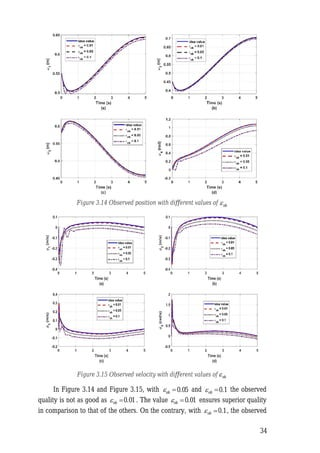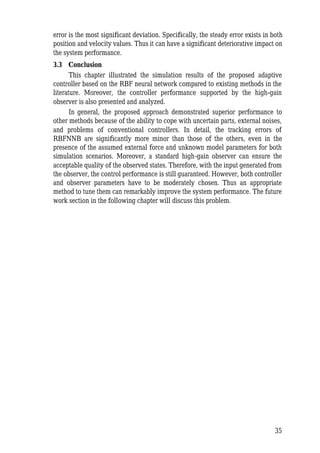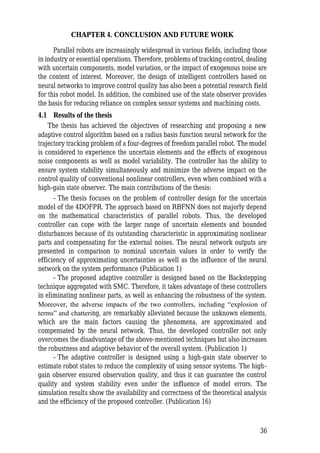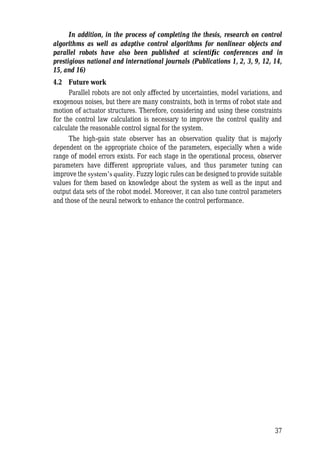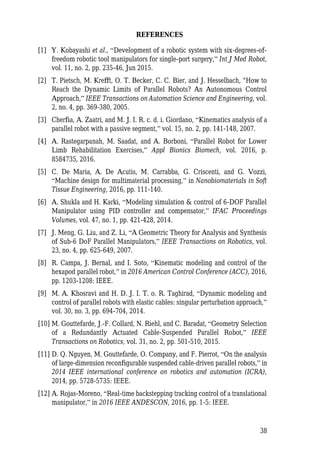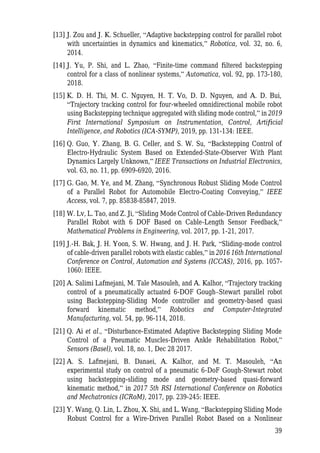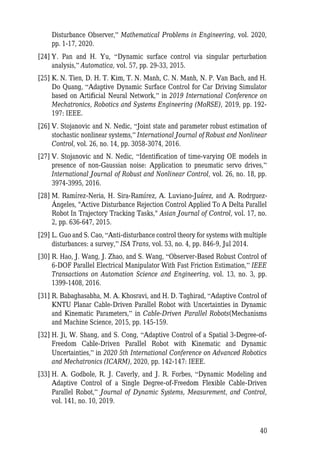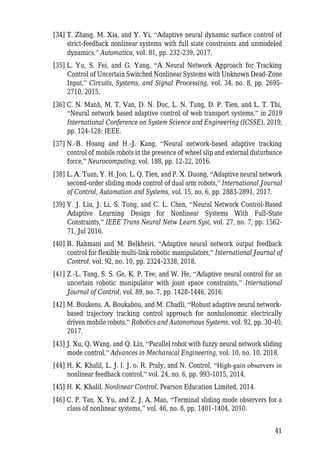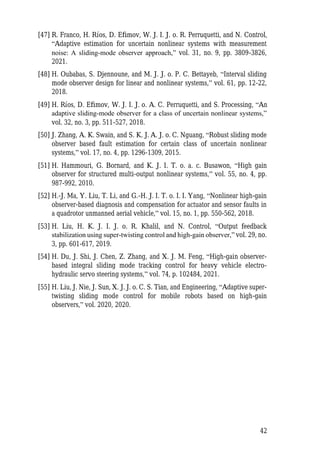Upcoming SlideShare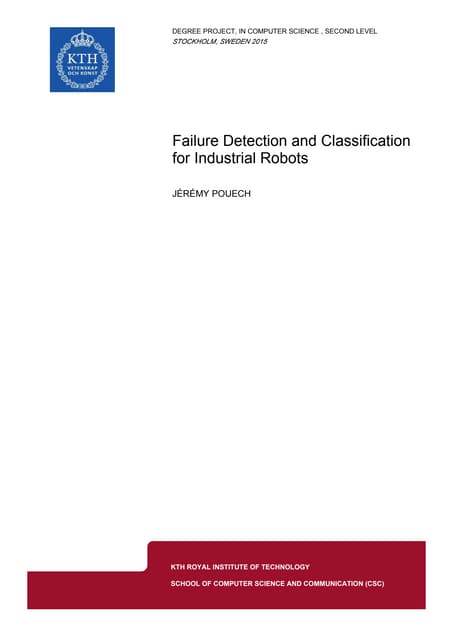Thesis_Report
1 of 49

### Design an adaptive controller and a state observer based on neural network for the 4DOF parallel robot.pdf

1. HANOI UNIVERSITY OF SCIENCE AND TECHNOLOGY MASTER THESIS Design an adaptive controller and a state observer based on neural network for the 4DOF parallel robot NGUYEN MANH CUONG Control Engineering and Automation Supervisor: Assoc. Prof. Nguyen Tung Lam School: School of Electrical and Electronic Engineering HA NOI, 2022
2. HANOI UNIVERSITY OF SCIENCE AND TECHNOLOGY MASTER THESIS Design an adaptive controller and a state observer based on neural network for the 4DOF parallel robot NGUYEN MANH CUONG Control Engineering and Automation Supervisor: Assoc. Prof. Nguyen Tung Lam School: School of Electrical and Electronic Engineering HA NOI, 2022 Supervisor’s Signature
3. C NG HÒA XÃ H I CH T NAM Ộ Ộ Ủ NGHĨA VIỆ Độ ậ – ự – ạ c l p T do H nh phúc B N XÁC NH NH S A Ả ẬN CHỈ Ử LUẬN VĂN THẠC SĨ H và tên tác gi ọ ả luận văn : Nguyễ ạnh Cườ n M ng tài lu Đề ận văn: Thiết k b ế ộ điều khi n thích nghi và b quan sát tr ng thái ể ộ ạ d a trên m ự ạng nơ ron cho robot song song bốn b c t do ậ ự (Design an adaptive controller and a state observer based on neural network for the 4DOF parallel robot) Chuyên ngành: K thu u khi n và T ng hóa ỹ ậ ề t Đi ể ự độ Mã số SV: 20202916M Tác giả, Người hướng d n khoa h c và H ẫ ọ ội đồng ch m lu ấ ận văn xác nhận tác gi a ch a, b sung lu n h p H ng ngày ả đã sử ữ ổ ận văn theo biên bả ọ ội đồ 04/05/2022 v i các n i dung sau: ớ ộ - nh s i chính t m c Chỉ ửa lỗ ả và đánh đề ụ - nh s a các ký hi u và b sung danh m vi t Chỉ ử ệ ổ ục từ ết tắ Ngày 07 tháng 05 năm 2022 Giáo viên hướ ẫ ả ận văn ng d n Tác gi lu CH CH H NG Ủ Ị T Ộ Ồ I Đ
4. 1 TABLE OF CONTENT CHAPTER 1. OVERVIEW................................................................................. 1 1.1 .................. 1 The four degrees of freedom parallel robot (4DOFPR) model 1.2 ................................... 2 Trajectory tracking controllers and state observers 1.2.1 controllers.................................................... 2 Trajectory tracking 1.2.2 State Observers ........................................................................... 5 1.3 Conclusion.................................................................................................. 7 CHAPTER 2. DESIGN AN ADAPTIVE CONTROLLER AND A STATE OBSERVER FOR THE FOUR DEGREES OF FREEDOM PARALLEL ROBOT ................................................................................................................. 8 2.1 4DOFPR......................................................... 8 Mathematical model of the 2.1.1 ......................................................................... 9 Kinematic model 2.1.2 ......................................................................... 10 Dynamic model 2.2 ................................................................ 10 Controller design for 4DOFPR 2.2.1 Backstepping aggregated with SMC (BASMC)....................... 10 2.2.2 -based (RBFNNB) adaptive controller........................ 13 RBFNN 2.2.3 -gain observer for the adaptive controller......................... 17 High 2.3 Conclusion................................................................................................ 20 CHAPTER 3. SIMULATION RESULTS........................................................ 22 3.1 Results of the RBFNN based adaptive controller (RBFNNB) ................ 22 3.2 Simulation results of the adaptive controller using the high-gain state observer................................................................................................................ 27 3.3 Conclusion................................................................................................ 35 CHAPTER 4. CONCLUSION AND FUTURE WORK................................. 36 4.1 Results of the thesis.................................................................................. 36 4.2 Future work .............................................................................................. 37 REFERENCES................................................................................................... 38
5. 2 LIST OF TABLES Table 3.1 Reference trajectory parameters .......................................................... 22 Table 3.2 Control parameters............................................................................... 23 Table 3.3 Trajectory reference parameters .......................................................... 28
6. 3 LIST OF FIGURES Figure 1.1 Parallel robot applied in the car motion simulator ............................... 2 Figure 1.2 Parallel robot applied in rehabilitation system  ............................... 2 Figure 2.1 (a) Robot coordinate; (b) Vector diagram of 4DOFP........................... 8 Figure 2.2 Structure of BASMC controller.......................................................... 12 Figure 2.3 RBFNN structure................................................................................ 14 Figure 2.4 Structure of the adaptive controller .................................................... 15 Figure 3.1 ...................................................................................... 23 External force Figure 3.2 Motion trajectory of p ....................................................................... 24 Figure 3.3 Tracking error of p ............................................................................ 25 Figure 3.4 Approximated values.......................................................................... 26 Figure 3.5 Motion trajectory of q ........................................................................ 27 Figure 3.6 Tracking error of q ............................................................................. 27 Figure 3.7 Uncertain parts in the robot model ..................................................... 29 Figure 3.8 Observed values of q ......................................................................... 30 Figure 3.9 Observed values of q ......................................................................... 30 Figure 3.10 Observational error of q ................................................................... 31 Figure 3.11 Estimated values from RBFNN........................................................ 32 Figure 3.12 Robot’s trajectory ............................................................................. 33 Figure 3.13 Tracking error ................................................................................... 33 Figure 3.14 Observed position with different values of ob  ................................ 34 Figure 3.15 Observed velocity with different values of ob  ................................ 34
7. 4 LIST OF ABBREVIATIONS Abbreviation Definition 4DOFPR Four Degrees of Freedom Parallel Robot DOF Degrees of Freedom SMC Sliding Mode Control DSC Dynamic Surface Control RBFNN Radius Basis Function Neural Network BASMC Backstepping aggregated with Sliding Mode Control RBFNNB Radius Basis Function Neural Network- based DSCNN Dynamic Surface Control Neural Network
9. 2 Figure 1.1 Parallel robot applied in the car motion simulator Figure 1.2 Parallel robot applied in rehabilitation system  From the reference and analysis of the above scientific works, moreover, intending to reduce the computational complexity and redundant constraints while still ensuring the necessary motion, the thesis puts focus on the four degrees of freedom parallel robot platform with the movements of rotational and translational movements along the OZ axis, rotation in the OX axis and the OY axis. 1.2 Trajectory tracking controllers and state observers 1.2.1 s Trajectory tracking controller In robot control, especially in orbital tracking control problems, modern methods specially put focus on designing control algorithms capable of handling problems related to uncertainties, perturbations, and unknown structural components in the system model while still ensuring stability and tracking quality. The 4DOFPR parallel robot model is considered to be a model being commonly affected by nonlinear uncertain elements in practical applications, especially external forces acting in different directions on the system. The parallel structures are considered a nonlinear model in the control design field; therefore, a control issue has attracted significant attention in the scientific community. One of these designed methodologies for nonlinear control systems
10. 3 that the have been interested in is Backstepping technique as in , , , , and  in order to ensure the quality of trajectory tracking control. However, when uncertainties or unmodeled components exist in the system model, the “explosion of terms” phenomena adversely affects the control quality. Another prominent control method is sliding mode control (SMC) which has been widely used because of its robust characteristic as in , , and  when considering the existence of unknown elements. However, the chattering phenomenon generated by SMC controller is likely to demolish the system , as well as the the computational burden with the high order systems. Combining the two aforementioned controllers is an approach to improving control performance because it takes advantage of them. Then, the robustness characteristic is enhanced, and the computational cost is reduced as in , , , and . Nevertheless, the combined controller cannot cope with the chattering and “explosion of terms” phenomena. On the other hand, by taking advantage of the multiple sliding surface controller and Backstepping technique, dynamic surface control (DSC) has been proposed to address the problem “explosion of terms” in  and  by using a low-pass filter for each computation step. However, the errors of the low-pass filter in the DSC controller are a dilemma, majorly depending on a ﬁlter time constant and being proven by complex mathematical conditions in , which may correlate with the frequency of experimental devices. Alternatively, a more efficient method in this paper handling mathematics difficulty is utilizing a neural network to approximate virtual signals and alleviate the chattering phenomena. In control theory, noise components are commonly considered to be an inevitable part of the whole system, and analyzing noise is the key to finding a way that assists the 4DOFPR system to be more stable and accurate. To be more specific, stochastic disturbances are problematic, 4DOFPR system. impacting the In terms of non-Gaussian noises, the modified extended Masreliez Martin filter – constructed in  is an efficient approach to handle nonlinear systems when environmental disturbances influence the whole system. Besides, stochastic parameters have been taken into consideration in  by estimating stochastic nonlinear systems. By taking into cautious consideration published in  and , it is assumed that some stochastic disturbances as to an unknown varying force from the input system act on actuators of the 4DOFPR system along the vertical direction because of body weight and moment disturbance as well as unknown parts. However, there have been several kinds of noises in external and internal stochastic disturbances because of all range elements , from frictions, vibrations, and changes of sudden forces to the shift in environmental conditions, which are considered uncertainties. In this thesis, we assume that the 4DOFPR is the model prone to the impact of stochastic uncertainty elements. As mentioned above, for many conventional nonlinear controllers such as SMC or Backstepping, there have been drawbacks in improving control performances when it is challenging to identify the accurate model because of the
13. 6 limited by cost and physical constraints, so observers play an essential role in many applications. Commonly, linear observers and sliding mode observers are constructed for linear systems. However, the fact that the system contains nonlinear components, even uncertain components, it is challenging for these observers to guarantee the quality of observation and control of the system. Therefore, nonlinear observers have been researched and designed with specific structures and advantages , , , , , and . The most prominent and prevalent observers for the class of nonlinear systems are the high-gain observers , , the sliding mode observers , , , and extended observers. The four-degree-of- freedom parallel robot model is an object that contains highly nonlinear components and is also affected by noise and unknown components. Besides, although it is robust to noise, the sliding observer has the well-known chattering phenomenon. Therefore, extended or adapted observers or techniques were studied in , , , and  to improve the quality and handle this phenomenon. However, this also increases the complexity of the computation and implementation of these observables. Therefore, due to its ability to minimize the impact of model variation and uncertainty components along with a simple design structure and ease of implementation in practice, the high-gain observer is chosen to design for the robot object in the thesis. In , , and , an overview of high-gain observers was introduced for nonlinear systems and feedback control systems. In addition, the observer design structure for the multi-input, multi-output nonlinear systems was also constructed in  in two approaches, including a general method for the observed nonlinear system and the other which structure is decomposable into linear and nonlinear parts. It can be seen that the high-gain observer has the advantage of observing systems with high-order nonlinear components as well as with disturbances , , and . Typically with the Quadrotor drone , the high-gain nonlinear observer was used to monitor, analyze, and compensate for the actuator s deviation and the measurement errors. In addition, the controller ’ designed in  reduced the use of sensors and actuators when using an observer. When using a high-gain observer with feedback controllers for a nonlinear system, especially a sliding mode controller, the system’s overall stability was ensured even if the system contains uncertain components . Besides, the study  proposed a structure of the high-gain observer combined with control law for an electro-hydraulic servo steering system because of its complex structure and variable load. The automatic steering system plays a decisive role in the stability of self-driving car systems. The flexible combination of the observer and the controller not only reduces the complexity of using the sensors but also ensures the stability of the system. In addition, adaptive controller using a high-gain an observer was also designed for the mobile robot to solve the problem of orbital tracking .
14. 7 From the reference, analysis, and evaluation, the high-gain observer will be constructed for the neural network-based adaptive controller for the uncertain model of 4DOFP affected by exogenous noise. Thanks to the ability to quickly converge as well as handle mathematical model errors, the high-gain observer can ensure observation quality to guarantee the quality of the trajectory tracking control problem. The observer design and its use for the adaptive controller will be presented in Chapter 2, along with the results accompanied by the analysis and evaluation of the whole system quality in Chapter 3. 1.3 Conclusion In Chapter 1, an overview of the four-degree-of-freedom parallel robot was presented along with various applications in several fields. From the study and analysis of recent studies on nonlinear controllers in solving the trajectory tracking problem, an adaptive controller based on a radial neural network is proposed to solve the problem of robot model uncertainty as well as the disadvantages of model-based controllers. Prominent representatives of state-observer sets were also reviewed. From the tracking control problem for a parallel robot model with four degrees of freedom combined with the analysis of related scientific publications, the scope and research objectives of the thesis have been proposed and presented.
15. 8 CHAPTER 2. DESIGN AN ADAPTIVE CONTROLLER AND A STATE OBSERVER FOR THE FOUR DEGREES OF FREEDOM PARALLEL ROBOT This chapter presents the design of an adaptive controller and a state observer for a four-degree-of-freedom parallel robot. First, the kinematics and dynamics equations are described to serve as a premise for controller and observer design. Then, an adaptive controller will be designed using the combination of the Backstepping controller and the sliding mode control technique to take advantage of the two controllers for nonlinear systems. Next, the radial radius neural network and the update rule designed based on the Lyapunov stability criterion are constructed to approximate the uncertain components to overcome the disadvantages of the above controllers and enhance the control quality for the robot system. Finally, a high-gain state observer is used to reduce the complexity of using sensors to measure the motion velocity quantities of the robot. 2.1 Mathematical model of the 4DOFPR (a) (b) Figure 2.1 (a) Robot coordinate; (b) Vector diagram of 4DOFPR. This section describes the robot’s kinematic and dynamic models followed by a global problem in the control design procedure. Figure 2.1 (a) depicts the overall system and system coordinates. The global coordinate is chosen as in Figure 2.1 (b). System states are rotational angular about Ox, Oy, Oz axes, and translational position along the vertical axis of the upper platform’s panel. Robot movements are performed by simultaneous vertical motions of three pistons combined with the rotation of a rotating shaft attached below the base platform. The kinematic model demonstrates a transformation between the piston’s movement and P ’s position, shown in the following subsection. Model-based control of robots requires a computationally efficient formulation of motion equations achieved by expressing the dynamic model in terms of a set of independent joint coordinates, referred to as minimal coordinates. Minimal coordinates formulations have been widely used for nonlinear motion control of robot manipulators. To this end, the joint coordinates of a robot
16. 9 manipulator that is subjected to kinematic loop constraints are split into a set of dependent and independent coordinates 2.1.1 Kinematic model The system state vector is denoted as   , , , T z p    = p , where z p is vertical position and  ,  , and  are rotational angular about and OX, OY, OZ axes, respectively. Besides, vector   1 2 3 T l l l  = q describes the piston’s lengths and OZ rotational angular with i l is the piston length i ( 1,2,3) i = . c is the distance between origin O and base platform’s A O , while a and b are the radius of upper and base platforms, respectively cording to the vector diagram, a . Ac vector equation of 4DOF is computed as PR : , i i i A i A A B =OP+PB -O A -OO (2.1) where 1,2,3 i = . The coordinates of A1, A2, A and 3 in the local coordinate 1 1 1 PX YZ associated with the base panel’s center are 1 sin( ) cos( ) 0 , 6 6 T base A a a     =       2 0 0 , T base A a = − and 3 sin( ) cos( ) 0 6 6 T base A a a     = −     . Assuming that a b = , the coordinates of B1, B2, B and 3 in the local coordinate A O XYZ associated with the upper panel’ center are 1 sin( ) cos( ) 0 , 6 6 T upper B a a     =       2 0 0 , T upper B a = − and 1 sin( ) cos( ) 0 6 6 T upper B a a     = −     . Besides, the cent points of the base and upper er panel are   0 0 T A O c = and   0 0 T de z p p = in the global axis, respectively. Then, we obtain fixed coordinates of i A and i B are . i A ibase A A T A O = + , and . i P ibase de B T B p = + with cos sin 0 sin cos 0 0 0 1 A T     −     =      and cos cos cos sin sin cos sin sin sin cos cos sin cos sin cos cos sin sin sin cos sin sin cos sin sin cos sin cos cos P T                              − +     = + −     −   . Finally, the length of the robot’s legs is computed as 2 , i l = i i A B (2.2) where i i B A = − i i A B . From (2), q can be computed from p.
17. 10 2.1.2 Dynamic model Commonly, to achieve more precise movement, the controller takes the dynamic model, which additionally considers the kinetics energy of the system, into account. Therefore, the elements containing forces, acceleration, mass, inertia, and other system parameters are put effort to accurately identified. It is evident that with the controller designed based on a dynamic model, the more reliable the system is, the better control quality can obtain. Nevertheless, it is challenging to identify these parameters exactly, and thus, there has been errors between the actual and mathematical model. Therefore, assuming that the dynamic robot model is a nominal model with bounded uncertain elements and noises. According to , the dynamic model of the robot based on Euler- the Lagrange form is described by , M(q,q F (2.3) in which 2 2 2 2 2 0 9 9 9 0 9 9 9 0 9 9 9 0 0 0 4 12 p py p p dc p p px p dc p p p dc m I m m m m m m I m m m m m m a m m I a                 + + +         + + + =       + +              M with 2 2 1 2 ( ), ( ), ( ), ( ), ( . ), ( . ), dc p px py m kg m kg m kg m kg I kg m I kg m and 2 ( . ) I kg m  are the mass of the covering of pistons, the mass of pistons, the mass of motors on the cylinders, the mass of the mobile panels, and the inertia of the mobile panels about , , Ox Oy Furthermore, 1 4 2 4 15 4 1 0 0 0 5 0 0 0 2 0 0 0 0 0 0 0 0 py px I l I a a l         =           C and   2 1 1 1 0 3 T p m m g   =     + D with 2 9.8( / ) g m s  is the gravity acceleration. 1 2 3 T F F F     =  F is defined as a control signal vector. 2.2 Controller design for 4DOFPR 2.2.1 Backstepping aggregated with SMC (BASMC) The controller designed by combining the two controllers can ensure that the robot tends to track the desired trajectory with the identified mathematical model. By combining Backstepping technique and SMC method, the controller takes the
18. 11 advantage of the two control algorithms, which means it can eliminate the system’s nonlinear elements and increase robust behavior to external noises To facilitate the control design procedure, initially, assuming that , , M C and D are accurately known, which means all the information concerning the robot system is clearly identified. Therefore, the controller is designed under ideal conditions with all information available. Subsequently, a reference for trajectory the dynamic model   1 2 3 ( ) ( ) ( ) ( ) ( ) T d d d d t l t l t l t t  = d q is computed from the robot’s reference trajectory is defined as and   ( ) ( ) ( ) ( ) ( ) T zd d d d t p t t t t    = d p using the forward kinematic equations. To clearly describe the tracking process, in the beginning, the difference between q and d q in each control period is denoted by . = − 1 d ξ q q (2.4) With the tracking error vector 1 ξ , the control objective is now equivalent to force 1 ξ tend to vicinity of zero. A virtual control which is chosen as the derivative the of q, which satisfies the following Lyapunov candidate function 1 1 . 2 V = T 1 1 ξ ξ (2.5) From a first-order derivative of 1 V , it is straightforward to show that 1 V − 1 1 1 d (2.6) the equation of q has a form of = − d 1 1 α q (2.7) Next, the the vector depicting between error q and α is defined as = − 2 ξ q (2.8) Alternatively, we can write , = + 2 q and 1 V turns into 1 , V − T 1 2 1 1 1 ξ k ξ (2.9) where 11 12 13 14 0 0 0 0 0 0 0 0 0 0 0 0 k k k k       =       1 k is a diagonal positive definite matrix. In addition, 2 ξ is also considered as a sliding manifold to compute the system control input.
19. 12 Figure 2.2 Structure of BASMC controller Figure 2.2 illustrates the structure diagram of the BASCM controller. The tracking error is considered as an input for the Backstepping controller, and the virtual control signal is calculated. In fact, the physical value of this signal is the ideal velocity of the robot’s lengths and the rotational about Oz axis. After the desired control signal is derived, the virtual signal error is fed into the sliding mode control to calculate the control signal for the system. Taking derivative of 2 ξ results in = − + − 2 ξ (2.10) Based on the Backstepping technique’s design steps, we propose the second Lyapunov candidate function to calculate the system input. The system’s global stability is taken into account according to this function 2 1 1 . 2 V V = + T 2 2 ξ ξ (2.11) Taking derivative of 2 V leads to 2 ( ( V − + − + − T T -1 -1 1 2 2 1 2 1 1 1 2 ξ k ξ ξ M F M Cq (2.12) The control signal F compris two parts: an equivalent control signal and es a switching control signal having the form of the SMC controller. The equivalent control eq F is chosen as follows = + − − eq 1 F Cq (2.13) which holds the system state on the defined sliding manifold. Besides, the switch control sw F drives the system state to the sliding surface ( ( ) ), sign =− + sw 2 2 3 2 F M k ξ k ξ (2.14) where 21 22 23 24 ( , , , ) diag k k k k = 2 k and 31 32 33 34 ( , , , ) diag k k k k = 3 k are diagonal positive definite matrices. One of the main factors causing the chattering phenomena is the presence of the signum Commonly, to decrease the impact of this issue, function. the satlins function is , and the switching control signal (2.14) selected is transformed into ( ( ) ), sat =− + sw 2 2 3 2 F M k ξ k ξ (2.15) Eventually, the control signal is obtained by
20. 13 = + eq sw F F F . (2.16) However, there will be a trade-off between the robustness of the system and the smoothness of the control signal. In addition, this phenomenon can be eliminated only in a certain range using this method. Theoretically, the chattering phenomenon is majorly caused by the existence of unmodeled dynamic elements in the systems. The RBFNN can estimate appropriate values for unmodeled elements, thus reducing impacts of the chattering phenomenon. the Substituting (2. ) (2.12), 16 into 2 V turns into 2 ( ) . V sat − − T T 1 1 1 2 2 2 2 3 2 ξ ξ k ξ ξ k ξ (2.17) where 1 k , 2 k , and 3 k are chosen as above, 2 V satisfied the Lyapunov which stability standard. Remark 1. BASMC controller is constructed based on the Backstepping technique is ; therefore, the phenomenon called “explosion of terms” caused by iteratively and incrementally taking derivative of the virtual control signal (2.7). Especially when the system contains uncertain elements such as discontinuous functions, this calculation can have an adverse effect on system performance. Additionally, even though the satlins function in (2.15) has ability to reduce the the chattering’s impact, there will be a trade off in the system’s robust - characteristic since this model-based controller cannot capture all the dynamics in the 4DOFPR model, which is often subjected to uncertainties, noises, and external forces. 2.2.2 -based (RBFNNB) adaptive controller RBFNN In practice, it is difficult to precisely identify , , M C D, and external disturbances d τ . Therefore, with -based a model controller such as BASMC, the control law only uses nominal values of these matrices. Hence, BASMC may not meet the tracking quality because of the “explosion of terms” phenomena accompanied by the chattering problem. This section proposes a controller which can solve the problems stemming from uncertain elements and external disturbances. , , M C and D are considered as the values of parameter actual system matrices. Besides, , , M C and D are their nominal values. Then, ΔM , ΔC , and ΔD are denoted as the differences between ideal and nominal values of these uncertainties. Without loss of generality, it can be shown that , , = + = + M M ΔM C C ΔC and = + D D ΔD, the robot system (2.3) is transformed into + + = + − + + d M (2.18) To facilitate the control design steps, (2. ) is rewritten 18 as = − + + − + + d (2.19)
21. 14 Finally, system dynamic equations turn into = − + + (2.20) where 4 1 ( ( )) . R  = − + +  -1 d δ M τ ΔM ΔD Conducting the same procedure in the previous section, with the defined as uncertainties, 2 ξ becomes = − + + − 2 ξ (2.21) The iterative calculation of α causes “explosion of terms”, therefore, to overcome this problem, propos an RBFNN and ing to estimate the uncertainties provide the appropriate value for the derivative of α. Moreover, estimated values generated by RBFNN for the un parts can significantly reduce certain dynamical chattering impacts. The RBFNN has the ability of online learning to approximate highly-nonlinear functions, and thus, the controller does not require full or even any prior accurate knowledge about the system. Assuming that α is unknown and considering 1 2 3 T        = = −   σ δ α = − + + 2 ξ (2.22) The control signal = + eq sw F F F with eq F and sw F s are rewritten a ˆ, = + − − eq 1 F Cq ξ Mσ (2.23) ( ( ) ). sign = − + sw 2 2 3 2 F M k ξ k ξ (2.24) respectively. In (2. ), 23 4 1 ˆ R   σ is an out vector of the neural network. In addition, σ is denoted as the idea value for σ̂ , and thus, RBFNN is designed to guarantee . σ̂ . approaches σ with arbitrarily small error or estimate an appropriate value an for σ . to ensure the system’s stability Figure 2.3 RBFNN structure Figure 2.3 shows the RBFNN’s structure with three layers: an input layer, a hidden layer, and an output layer. The neural structure is constructed with , = + T σ W h ε (2.25) ˆ ˆ , T = σ W h (2.26)
22. 15 where ε is approximation error and   ε with  is an arbitrarily small positive constant. W and Ŵare ideal weight and updated weight matrices, respectively. Additionally, ˆ W W is considered as the error weight matrices. RBF function is the employed to calculate output of the hidden layer as follows 2 2 exp , 2   − = −      x h      (2.27) where the system state q is chosen as an input vector,      is a center point, and  presents the width value of RBF function the h . Substitute the control signal in (2.23) (2.24) into (2.22) we obtain and ( ) sign − + 2 2 2 3 2 ξ k ξ k ξ (2.28) Adaptive law for RBFNN’s weight is chosen as ˆ ( )  = − T 2 2 W Γ hξ ξ W , (2.29) where n n R   Γ positive definite matrix with is a n being node number of the hidden layer, a learning rate parameter is chosen in (0,1) . Figure 2.4 Structure of the adaptive controller The structure of the proposed controller based on RBFNN is shown in Figure 2.4. In comparison to BASMC structure, the RBFNN with the input of ’s robot states is constituted to estimate the appropriate values for σ̂ with online weight updated law the adaptive controller. in The second Lyapunov candidate function is reselected as 2 1 1 1 ( 2 2 V V tr = + + T 2 2 ξ ξ W (2.30) and 2 V becomes 2 V 1 2 2 (2.31) Substituting (2. ) (2.31) y lds 28 into ie
23. 16 2 ( ) ( ) ( V sign sign tr = − − − + + = − − − + + 1 2 1 2 2 3 2 T T T T T 1 1 1 2 2 2 2 3 2 2 2 T T T T 1 1 1 2 2 2 2 3 2 2 2 ξ k ξ ξ k ξ ξ k ξ ξ ε ξ W ξ k ξ ξ k ξ ξ k ξ ξ ε W (2.32) With the updated law (2.29), 2 V is rendered as 2 V 1 1 1 2 2 2 2 3 2 2 2 (2. ) 33 Applying the Cauchy-Schwarz inequality results in 2 ( F F F tr W (2.34) It can be shown that 2 2 2 2 2min 3min 1 1 ( 4 2 N F F F N F F F V k k     − − − + + − − 1 1 1 2 2 2 3 2 2 T 1 1 1 2 2 2 2 2 ξ k ξ ξ ξ ξ ξ W ξ W W (2.35) where 2min k and 3min k are the minimum values of 2 k and 3 k . If the bounded condition in the following inequality is satisfied 2 2min 3min 1 4 N F k k   + −  2 W ξ (2.36) then 2 1 2 F F V 1 1 1 2 (2.37) and the system is global asymptotical stability. Furthermore, the updated law of the network is designed and proven based on Lyapunov standard, and the the convergence of the method is ensured. Remark 2. With the uncertain model of the system, the RBFNN plays a key role in estimating the appropriate values for the uncertainties to ensure control quality. ˆ (0) W is an initial value of a weight matrix W , and it is chosen based on the scope of the input data, especially the system’s states. Moreover, the updated law is to calculate an appropriate value for W, which is proven based on the Lyapunov standard to ensure convergence. In the radial basis function activation h of the hidden layer:      is the center point, and η lue, represents the width va and they should be moderately chosen based on knowledge concerning the system and input and output data. Center point The      ha impact on the sensitivity of s an the RBF function to , and it opts range of the system the input vector’s scope in the state vector q. Besides, η expresses the covering scope of the network input. If these parameters are chosen inappropriately, the RBF will not be effectively mapped, and RBFNN will not take effect.
24. 17 2.2.3 -gain observer for the adaptive controller High Typically, a combination of measurement and data processing from sensors is synthesized to obtain robot states position and velocity values . However, in ’ many circumstances, the construction of the sensor system and the calculation of the necessary values are significantly complicated and costly to satisfy the acceptable accuracy due to the influence of environmental conditions, noises, and sensor quality. Therefore, the observer is constructed to estimate the system states, namely the velocity values of the parallel robot system. On the other hand, a four- degree-of-freedom parallel robot system is fast responsive; hence, the observed velocity signal requires a fast convergence rate with the actual velocity value. Thus, the high-gain observer is selected due to its advantages of convergence speed, as well as resistance to model errors , , and .  To observer design process, the variables are defined as facilitate the auxiliary follows       1 2 3 1 2 3 4 1 2 3 1 2 3 4 , T T T T l l l l           = =    = =     ω      (2.38) Then the system states are denoted by     T T x q q   = = , the ideal dynamic model of the robot is rewritten as ( ) ( ) ( ) ( ) , ,     = =   − −     q q x M q q q q (2.39) with assuming that the control signal F . is able to ensure the system’s stability From that, the ideal values for the observers are generated by ( ) 1,2,3,4 i i i i   =    =   (2.40) with , ( 1,2,3,4) i i i   = are the ideal values of , 3,4) i i l l , respectively. Next, defining ˆ ˆ , ( 1,2,3,4) i i i   = as the high-gain observer outputs. The equations of the high-gain observer are described by ( ) ( ) 1 1 2 2 ˆ ˆ, , , , , 1,2,3,4 i i i i i i i ob i i i i i i ob k j e e k f q q F j e f q q F e i        = + = +     = =   (2.41) with 1 2 ˆ , , 0,0 i i i i i ob e k k    = −   The parameters 1 2 , i i k k to satisfy are chosen that 2 1 2 i i s k s k + + polynomial. is the Hurwitz In (2.41), the ideal dynamic model is taken into account to update the robot’s obser . However, uncertain components exist evermore in the system ver’s outputs model. A considerable advantage of a high-gain observer is that it can minimize
25. 18 the influence of variable parameters and uncertainty components in the model. Assuming that the dynamic . matrices are nominal values containing uncertainties Then (2.41) is rewritten as ( ) ( ) 1 1 2 2 ˆ ˆ, , , , , 1,2,3,4 i i i i ii i i ob i i i ii i i ob k j e e k f q q F j e f q q F e i        = + = +     =   (2.42) in which ( 1,2,3,4) i f i = are the nominal values experienced the effect of uncertain parts and model errors of i f . Additionally, assuming that the nominal model 1 2 3 4 T f f f f   =   f s sati fies ˆ L M −  − + f f x x (2.43) with L and M n-negative numbers. are no Then, let   T = x ω      and ˆ ˆ ˆ T   =   x ω      . The error between x and x̂ are defined by 2 ˆ ( , , , ) i i i ob i i i i i ob k k f e            −       − −     x q q q q F (2.44) From (2. ), (2.41), and (2.42), the equation (2.44) is transformed into 40 ob x (2.45) where   =     12 12 21 22 A A A A A with 11 11 11 11 0 0 0 0 0 0 0 0 0 0 0 0 ob ob ob ob k k k k       −       −     =   −       −     11 A , 1 0 0 0 0 1 0 0 0 0 1 0 0 0 0 1       =       12 A , 12 2 12 2 12 2 12 2 0 0 0 0 0 0 0 0 0 0 0 0 ob ob ob ob k k k k       −       −     =   −       −     21 A , 0 0 0 0 0 0 0 0 0 0 0 0 0 0 0 0       =       22 A ,
26. 19 Matrice 0 0 0 0 0 0 0 0 0 0 0 0 0 0 0 0 1 0 0 0 0 1 0 0 0 0 1 0 0 0 0 1             =               B , and ˆ ( , ) = − ob δ f q q . To more explicitly present an ability to cope with uncertain model of the high- the gain observer, s observer error by a vector caling the 3 1 2 4 5 6 7 8 T ob ob ob ob x x       =    ob γ yields ( ) ( ) ( ) ( ) 5 1 6 2 1 3 2 3 4 5 12 1 1 1 6 22 7 2 2 2 8 32 3 3 3 42 4 4 4 ob ob ob ob ob ob ob ob ob ob ob ob ob k x k x x k x x x k x x x x f x x x f x k x f k x f                   −    −     −      −   = =   − + −       − + −    − + − − + −  ob γ                 = +                          ob ob ob Dγ ε Bδ (2.46) with 11 21 31 41 12 22 32 42 0 0 0 1 0 0 0 0 0 0 0 1 0 0 0 0 0 0 0 1 0 0 0 0 0 0 0 1 0 0 0 0 0 0 0 0 0 0 0 0 0 0 0 0 0 0 0 0 0 0 0 0 0 0 0 0         −     −     −   −   =   −   −     −   −     D . Moreover, with ob  and the condition (2.43), the Lipschitz condition is converted into ob L M   + ob γ (2.47)
27. 20 The Lyapunov candidate function of the high-gain observer (2.42) for 4DOFPR is chosen as = T ob ob ob ob V γ P γ (2.48) where 0 =  T ob ob P P is the solution of + = − T ob ob P D D P I. Taking derivative of ob V 2 ob ob ob V   + T T ob ob ob ob ob γ γ P Bδ (2.49) Using the condition (2.47), (2.49) turns into 2 2 2 2 2 2 1 1 2 , 2 4 1 , 8 4 ob ob ob ob ob ob ob ob ob V L M M L M         +  − + + −  +   −    T T T ob ob ob ob ob ob ob ob ob ob ob ob ob ob ob ob ob γ γ P Bδ γ γ P B γ P B γ γ P B P B γ γ P B (2.50) According to Theorem 4.5 , in it can be concluded that ( )   / max e 0 , , nt ob ob m k M   −  ob ob γ γ , , 0 ob m n k   (2.51) This indicates that the observer s deviation from the actual value is a ’ bounded value. The smaller the value ob  , the faster this error decreases. Therefore, with a reasonably small value chosen of ob  , the observer can guarantee the convergence of the output value with the ideal value. However, from (2.51) it can be seen that the peaking phenomenon  causes the output value at the initial time to jump and reach large values when ˆ (0) (0)  x x , and this phenomenon can make the system becomes unstable. One of the methods to alleviate the impact of this phenomenon on the system is to saturate the controller output. Then, values beyond the response threshold of the system will be saturated; however, the control quality of the system will be affected. Remark 3. more explicitly demonstrate the To ability to cope with noises and uncertainties of the high-gain observer, the observer error x is scaled by ob γ . From (2.46), it can be seen that with the parameter 1 ob   , is moderately chosen the impact of ob δ on the system performance is reduced. However, if ob  is chosen with positive number, it can result in the unstable of the system. an infinitesimal Therefore, this parameter needs to be tuned based on the knowledge about the robot system input output data. and and 2.3 Conclusion In this chapter, a neural network-based adaptive controller and a high-gain state observer have been proposed and designed for the trajectory tracking control problem for the uncertain model of the four-degree-of-freedom parallel robot. The adaptive controller can ensure system stability even when the model contains
28. 21 parameter variation, uncertainties, and noise components. Furthermore, the proposed controller can minimize the effects of explosion of terms and “ ” chattering phenomena on the control quality of the system. In addition, the state observer used estimate the system states even when the model errors exist. can To solve the control problem First, design the Backstepping algorithm combined with sliding mode + control to solve the problem of tracking control for parallel robots with four degrees of freedom with the assumption of an ideal robot model. Conduct simulation to verify, evaluate, and analyze the advantages and disadvantages of this method. Based on the analysis, propose an adaptive controller to improve the control quality. Propose a neural network-based adaptive control law for a four-degree- + of-freedom parallel robot model. The algorithm is constituted on the basis of the BASMC controller and the radius basis function neural network. The RBFNN is capable of approximating the appropriate values for uncertain components while compensating for the adverse effects stemming from the phenomena of conventional nonlinear controllers and noises. Then, the control quality of the system is expected to be significantly improved. Furthermore, a high-gain state observer is also used to estimate the system states, minimizing the complexity of using and calculating these values from the sensor system.
30. 23 Table 3.2 Control parameters Control gains 1 2 3 8; 10; 10. k k k = = = RBFNN parameters Neural number: 50 n = ; 0.1  = ; ˆ (0) 0 = W ; 1 2 3 1 2 3 1 1 2 2 3 3 15 15 ; ( ( , , ) ( , , ) ; ( , , ) , , ) 15 5 l l l linspace r r n linspace r r n linspace r r n linspace r r r r n r r      =   =   = = −    = −    = −   =  =     − 1 2 3 ; 40. T l l l     =     =        (2.5,2.5,....,2.5) n n diag  = Γ Figure 3.1 External force Besides, system specifications include: a =b=0.25 (m) ; c=0.05 (m); ( ) z a =0.050 m , and nominal values of robot’s dynamic coefficients are p m =15(kg) ; 1 m =0.5(kg) ; 2 m =3(kg) ; dc m =3(kg) . Additionally, controller gains, as well as RBFNN parameters, are given in 3.2. Table The simulation scenario is conducted with nominal values of matrices , , M C and D; therefore, the system parameters are also nominal values. Moreover, the system is influenced by an external force in the vertical direction, whose value is described in Figure 3.1. The results are demonstrated by comparing the three controllers, including BASMC, Dynamic Surface Control Neural Network (DSCNN) in , and the proposed controller RBFNNB. With the external force acting on the system as well as inaccurate information of the system parameters, model-based controllers like BASMC cannot achieve acceptable control quality despite robust behavior their
31. 24 inherited from SMC, as in Figure 3.2. Moreover, -state errors exist in the steady system’s tracking process when CDS is affected by an external force. Specifically, the system is not likely to compensate for the deviations caused by an unexpected impact because of the lack of information in the system’s mathematical model On the other hand, with a self-learning capacity, the neural network-based controllers do not require any information related to the system. Therefore, these controllers can meet the control requirement although the mathematical is inaccurate or the system is affected by bounded s. As a external impact result, DSCNN and RBFNNB significantly perform than BASMC. To clarify this statement, the tracking errors of the system state p are shown in Figure 3.3, with maximum values belonging to BASMC and minimum ones of RBFNNB. Besides, in , DSCNN is demonstrated with the neural network ε and low-pass filter errors are ignored, and thus a learning rate is not considered in an adaptive law. Therefore, DSCNN ‘s convergence speed is slower than RBFNNB, which leads to D RBFNNB. SCNN ‘s tracking error being bigger than (a) Motion trajectory of z p (b) Motion trajectory of  (c) Motion trajectory of  (d) Motion trajectory of  Figure 3.2 Motion trajectory of p
32. 25 (a) Tracking error of z p (b) Tracking error of  (c) Tracking error of  (d) Tracking error of  Figure 3.3 Tracking error of p Initially, RBFNN takes time to estimate unknown information concerning the system. Hence, there is a fluctuation in the early period in responses of DSCNN and RBFNN when the estimated value considerably affects the control system’s quality, as in Figure 3.2 Figure 3.3. However, with the proper design, and RBFNNB takes advantage of RBFNN to simultaneously estimate appropriate values for uncertain parts and compensate for the disadvantages of model-based controllers, in this case, Backstepping and SMC. As a result, the RBFNNB’s fluctuation is not trivial. Moreover, when the system experiences abrupt changes of external forces at time instants 1s, 2s, and 4s, the system with RBFNNB an controller can promptly drive the system back to the stability states because RBFNN has almost immediately estimated the proper values (approximately 0.08s) as in Figure 3.4. It can be seen from Figure 3.4 that the solid lines depict the idea values which can guarantee the system’s stability and is unknown to the controllers. RBFNN is designed to estimate these values in the proposed controller, whose output is the dashed line. At the initial step, the neural network commences estimating the value for  with a convergence speed depending on the network structure and the chosen learning rate. By self-learning ability, RBFNN can
33. 26 generate unknown values that track the idea values even when sudden system information changes. (a) Approximated value of 1  (b) Approximated value of 2  (c) Approximated value of 3  (d) Approximated value of   Figure 3.4 Approximated values (a) Motion trajectory of 1 l (b) Motion trajectory of 2 l
34. 27 (c) Motion trajectory of 3 l Figure 3.5 Motion trajectory of q (a) Tracking error of 1 l (b) Tracking error of 2 l (c) Tracking error of 3 l Figure 3.6 Tracking error of q Finally, the tracking performance of q is shown in Figure 3.5 and Figure 3.6, and their trend tends to be similar to p . In general, the proposed controller RBFNNB gives the best performance compared to BASMC and DSCNN. 3.2 Simulation results of the adaptive controller using the high-gain state observer The high-gain state observer is to generate the input state signal for the adaptive controller designed in the previous chapter. Both the controller and the
36. 29 Figure 3.7 Uncertain parts in the robot model Besides, to verify the ability of insensitivity model errors and to uncertainties, assuming that the ideal model parameters p m =15(kg) ; 1 m =0.5(kg); 2 m =3(kg); dc m =3(kg) and those of nominal models are p m =25(kg) ; 1 m =1(kg) ; 2 m =10(kg); dc m =2(kg) . Furthermore, the system is assumed to be experienced uncertain parts, as in Figure 3.7. The uncertain elements, as well as the model variation, have adverse impacts on the quality of both controller and observer. However, as above-mentioned in the previous section, the RBFNNB adaptive controller appropriate can estimate the values for the controller.
37. 30 Figure 3.8 Observed values of q Figure 3.9 Observed values of q
38. 31 Figure 3.10 Observational error of q The control parameters are chosen in Table 3.2, and the observer parameters are selected 1 2 0.01; 1.0; 2.0( 1,2,3,4) ob i i k k i  = = = = . Because the objective is to estimate the robot’s velocity values and the robot position is still necessary for the observer, assuming that the initial position of the observer coincides with that of the robot. The observer outputs are given in Figure 3.8 and Figure 3.9. In Figure 3.8, it can be seen that the observed position tracks the actual values with minimal errors (approximate 10-4 ) with (m the robot’s legs and 10-4 (rad) of the angle  ) within the assumption is not different in the initial stage. However, this that it quantity is possibly measured to be used to calculate the observer outputs. Therefore, the comparison of position values is to demonstrate the high-gain performance. In addition, the observed velocity values in Figure 3.9 show that the observer can track the actual velocity. At the initial time, the velocities rapidly increase because the is different from that of the desired trajectory robot’s initial position . Thus, the controller generates the appropriate value to quickly steer the system to the reference. Moreover, it is evident that, although affected by the uncertain components and model variation, the velocity values of the robot legs and the angular velocity around OZ are still able to follow actual values with minor deviations within the acceptable range. Specifically, the observed velocity value errors are shown in Figure 3.10. In the beginning, the required observer will need time to calculate the appropriate value, hence the internal oscillation as shown in
39. 32 Figure 3.10. However, this time interval is also minimal (about 0.03s), and the convergence speed is fast, which is also one of the outstanding advantages of the high-gain observer. At the steady-state, the maximum deviation of the legs’ rate is only approximately 6.10-3 and that of the angular velocity is roughly (m/s), 5.10-4 (rad/s). In addition, it can be seen that there is a steady error phenomenon in the deviation values of the velocity observed because, according to (2.46), with a sufficiently small value of ob  , the observer is able to minimize the adverse effect of the mathematical model error. However, because of 0 ob   , this characteristic cannot eliminate errors entirely, but the parameter of the observer will be selected so that the deviation is kept in an acceptable range. From the afore analysis, it can be seen that the high-gain observer can ensure fast convergence speed and simultaneously minimize the impact of uncertainty components and model noise. With the ability to approximate the wide ranges of uncertainties, combined with the high-gain outputs, RBFNN still ensures that it can generate suitable values tracking ideal values for unknown parts to guarantee the system stability, as in Figure 3.11. Therefore, the control quality, as well as tracking performance, are ensured in Figure 3.12. Thus, the robot states can track their references with minor tracking errors (about 1,2.10-3 (m)) in Figure 3.13. Besides, there is also a steady error phenomenon occurring in the observer as . evaluations mentioned above However, with the 4DOFPR, the errors are acceptable. Figure 3.11 Estimated values from RBFNN
40. 33 Figure 3.12 Robot’s trajectory Figure 3.13 Tracking error To more extensively demonstrate the ability to alleviate the influence of model errors, different values of ob  are chosen. It can be seen that the minor ob  is, the better performance the system can achieve. However, if it is determined to be extremely small, peaking phenomena will affect the system, leading to  instability.
41. 34 Figure 3.14 Observed position with different values of ob  Figure 3.15 Observed velocity with different values of ob  In Figure 3.14 Figure 3.15, with and 0.05 ob  = and 0.1 ob  = the observed quality is not as good as 0.01 ob  = . The value 0.01 ob  = ensures superior quality in comparison to that of the others. On the contrary, with 0.1 ob  = , the observed
42. 35 error is the st significant deviation. Specifically, the steady error exists in both mo position and velocity values. Thus it can have a significant deteriorative impact on the system performance. 3.3 Conclusion This chapter illustrated the simulation results of the proposed adaptive controller based on the RBF neural network compared to existing methods in the literature. Moreover, the controller performance supported by the high-gain observer is also presented and analyzed. In general, the proposed approach demonstrated superior performance to other methods because of the ability to cope with uncertain parts, external noises, and problems of conventional controllers. In detail, the tracking errors of RBFNNB are significantly more minor than those of the others, even in the presence of the assumed external force and unknown model parameters for both simulation scenarios. Moreover, a standard high-gain observer can ensure the acceptable quality of the observed states. Therefore, with the input generated from the observer, the control performance is still guaranteed. However, both controller and observer parameters have to be moderately chosen. Thus an appropriate method to tune them can remarkably improve the system performance. The future work section in the following chapter will discuss this problem.